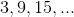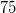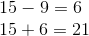## Example Questions

### Example Question #2 : Nth Term Of An Arithmetic Sequence

Given the sequence of numbers:

1, 5, 9, _ , _ , 21 ....

What are the two missing terms of the arithmetic sequence?

13, 16

12, 18

13, 17

14, 16

14, 17

13, 17

Explanation:

The sequence is defined by a= 4n – 3  for such n = 1,2,3,4....

### Example Question #3 : Nth Term Of An Arithmetic Sequence

What is the next term in the following sequence?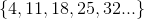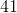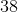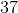Explanation:

What is the next term in the following sequence?This is an arithmetic sequence with a common difference of. To find the next term in an arithmetic sequence, add the common difference to the previously listed term: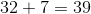### Example Question #4 : Nth Term Of An Arithmetic Sequence

Find the sixth term in the following number sequence.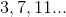Explanation:

This question can be answered by analyzing the sequence provided and determining the pattern. The first term is, and the second term is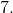The third term is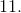Thus,has been added toin order to obtain, andhas been added toin order to obtainThis shows thatis added to each preceding term in the sequence in order to obtain the next term. The complete sequence from terms one through six is shown below.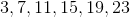Thus, the sixth term is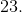### Example Question #5 : Nth Term Of An Arithmetic Sequence

What is the next term of the series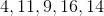?Explanation:

Begin by looking at the transitions from number to number in this series:

Fromto: AddFromto: SubtractFromto: AddFromto: SubtractFrom what you can tell, you can guess that the next step will be to add. Thus, the next value will be.

### Example Question #21 : Sequences

What is the next term in the following sequence: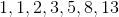?Explanation:

This sequence is a little tricky. Notice that the second element is equal to the first. After that, the third is equal to the sum of the first two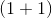, next the fourth is equal to the sum of the second and the third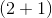, and the same continues for each element after this. Thus, the next element in the series will be equal to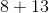or.

### Example Question #22 : Sequences

What is the next term in the sequence?